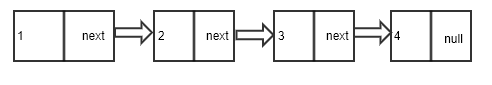RK Founder at HackersFriend Updated Jan. 8, 2020, 10:54 p.m. ⋅ 1084 views

# Find Length of a Linked List

We can find the length of a linked list by initializing a counter to zero and incrementing it for every node of linked list. We will traverse the linked list from start and go until we reach the end of it.In the above linked list, we can see there are 4 nodes and hence, the legth of this linked list would be 4.

Let's implement the appraoch to calculate the length of a linked list.

``````#include<bits/stdc++.h>
using namespace std;

struct node
{
int data; // holds the data
node* next; // hold the pointer to next node
};

{
// adds new node into the LL in the end
// takes the pointer to head of LL as arg
// and the data which is to be added

// create a new node to add in LL
node* new_node = new node();

// pass the data of arg to new node
new_node->data = data;
// set the next to null as its last node.
new_node->next = NULL;

// set it to point to head of LL

// reach the last node
while(temp->next != NULL)
{
temp = temp->next;
}

temp->next = new_node;
}

{
int counter = 0;
{
counter ++;
}

return counter;

}

int main()
{
cout<<"Total nodes in LL are "<<countNodes(head)<<endl;
return 0;
}
``````
``Total nodes in LL are 4``Join the community of 1 Lakh+ Developers